SLAS476H March   2006  – June 2017

PRODUCTION DATA.

1. Features
2. Applications
3. Description
4. Revision History
5. Pin Configuration and Functions
6. Specifications
7. Detailed Description
1. 7.1 Overview
2. 7.2 Functional Block Diagram
3. 7.3 Feature Description
1. 7.3.1 DAC Section
2. 7.3.2 Power-On Reset
4. 7.4 Device Functional Modes
5. 7.5 Programming
8. Application and Implementation
1. 8.1 Application Information
2. 8.2 Typical Applications
1. 8.2.1 Loop-Powered 2-Wire 4-mA to 20-mA Transmitter With XTR116
2. 8.2.2 Using REF02 as a Power Supply for DAC8550
3. 8.3 System Examples
1. 8.3.1 Microprocessor Interfacing
9. Power Supply Recommendations
10. 10Layout
11. 11Device and Documentation Support
12. 12Mechanical, Packaging, and Orderable Information

• DGK|8
• DGK|8

## 6 Specifications

### 6.1 Absolute Maximum Ratings

over operating free-air temperature range (unless otherwise noted)(1)
MIN MAX UNIT
Supply voltage GND –0.3 6 V
Digital input voltage range GND –0.3 VDD + 0.3 V
Output voltage GND –0.3 VDD + 0.3 V
Junction temperature, TJ(max) 150 °C
Operating temperature, TA –40 105 °C
Storage temperature, Tstg –65 150 °C
Stresses beyond those listed under Absolute Maximum Ratings may cause permanent damage to the device. These are stress ratings only, which do not imply functional operation of the device at these or any other conditions beyond those indicated under Recommended Operating Conditions. Exposure to absolute-maximum-rated conditions for extended periods may affect device reliability.

### 6.2 ESD Ratings

VALUE UNIT
V(ESD) Electrostatic discharge Human-body model (HBM), per ANSI/ESDA/JEDEC JS-001(1) ±2000 V
Charged-device model (CDM), per JEDEC specification JESD22-C101(2) ±500
JEDEC document JEP155 states that 500-V HBM allows safe manufacturing with a standard ESD control process.
JEDEC document JEP157 states that 250-V CDM allows safe manufacturing with a standard ESD control process.

### 6.3 Recommended Operating Conditions

over operating ambient temperature range (unless otherwise noted)
MIN NOM MAX UNIT
POWER SUPPLY
VDD Supply voltage 2.7 5.5 V
DIGITAL INPUTS
DIN Digital input voltage SCLK and SYNC 0 VDD V
REFERENCE INPUT
VREF Reference input voltage 0 VDD V
AMPLIFIER FEEDBACK INPUT
VFB Output amplifier feedback input VO V
TEMPERATURE RANGE
TA Operating ambient temperature –40 105 °C

### 6.4 Thermal Information

THERMAL METRIC(1) DAC8550 UNIT
DGK (VSSOP)
8 PINS
RθJA Junction-to-ambient thermal resistance 206 °C/W
RθJC(top) Junction-to-case (top) thermal resistance 44 °C/W
RθJB Junction-to-board thermal resistance 94.2 °C/W
ψJT Junction-to-top characterization parameter 10.2 °C/W
ψJB Junction-to-board characterization parameter 92.7 °C/W

### 6.5 Electrical Characteristics

VDD = 2.7 V to 5.5 V, –40°C to 105°C range (unless otherwise noted)
PARAMETER TEST CONDITIONS MIN TYP MAX UNIT
STATIC PERFORMANCE(1)
Resolution 16 Bits
EL Relative accuracy Measured by line passing through codes –32283 and 32063 at VREF = 5 V, codes –31798 and 31358 at VREF = 2.5 V DAC8550 ±16 LSB
DAC8550B ±12
ED Differential nonlinearity 2.5 V ≤ VREF ≤ 5.5 V, 0°C ≤ TA ≤ 105°C ±1 LSB
4.2 V < VREF ≤ 5.5 V, -40°C ≤ TA ≤ 105°C ±1 LSB
2.5 V ≤ VREF ≤ 4.2 V, -40°C ≤ TA ≤ 0°C ±2 LSB
EO Zero-code error Measured by line passing through codes –32283 and 32063 ±2 ±12 mV
EFS Full-scale error Measured by line passing through codes –32283 and 32063 ±0.05% ±0.5% mV
EG Gain error Measured by line passing through codes –32283 and 32063 ±0.02% ±0.2% mV
Zero-code error drift ±5 μV/°C
Gain temperature coefficient ±1 ppm of FSR/°C
PSRR Power-supply rejection ratio RL = 2 kΩ, CL = 200 pF 0.75 mV/V
OUTPUT CHARACTERISTICS(2)
VO Output voltage range 0 VREF V
tSD Output voltage settling time To ±0.003% FSR, 1200h to 8D00h, RL = 2 kΩ, 0 pF < CL < 200 pF 8 10 μs
RL = 2 kΩ, CL = 500 pF 12
SR Slew rate 1.8 V/μs
Capacitive load stability RL = ∞ 470 pF
RL = 2 kΩ 1000
Code change glitch impulse 1 LSB change around major carry 0.1 nV-s
Digital feedthrough SCLK toggling, FSYNC high 0.1 nV-s
zO DC output impedance At mid-code input 1 Ω
IOS Short-circuit current VDD = 5 V 50 mA
VDD = 3 V 20
tON Power-up time Coming out of power-down mode, VDD = 5 V 2.5 μs
Coming out of power-down mode, VDD = 3 V 5
AC PERFORMANCE
SNR Signal-to-noise ratio BW = 20 kHz, VDD = 5 V, fOUT = 1 kHz,
1st 19 harmonics removed for SNR calculation
95 dB
THD Total harmonic distortion BW = 20 kHz, VDD = 5 V, fOUT = 1 kHz,
1st 19 harmonics removed for SNR calculation
–85 dB
SFDR Spurious-free dynamic range BW = 20 kHz, VDD = 5 V, fOUT = 1 kHz,
1st 19 harmonics removed for SNR calculation
87 dB
SINAD Signal-to-noise and distortion BW = 20 kHz, VDD = 5 V, fOUT = 1 kHz,
1st 19 harmonics removed for SNR calculation
84 dB
REFERENCE INPUT
VREF Reference voltage 0 VDD V
II(REF) Reference current input range VREF = VDD = 5 V 40 75 μA
VREF = VDD = 3.6 V 30 45
zI(REF) Reference input impedance 125
LOGIC INPUTS (2)
Input current ±1 μA
VIL Low-level input voltage 3 V ≤ VDD ≤ 5.5 V 0.3 × VDD V
2.7 V ≤ VDD < 3 V 0.1 × VDD
VIH High-level input voltage 3 V ≤ VDD ≤ 5.5 V 0.7 × VDD V
2.7 V ≤ VDD < 3 V 0.9 × VDD
Pin capacitance 3 pF
POWER REQUIREMENTS
IDD Supply current Normal mode, input code equals mid-scale, no load, does not include reference current, VIH = VDD, VIL = GND VDD = 3.6 V to 5.5 V 160 250 μA
VDD = 2.7 V to 3.6 V 140 240
All power-down modes,
VIH = VDD, VIL = GND
VDD = 3.6 V to 5.5 V 0.2 2
VDD = 2.7 V to 3.6 V 0.05 2
POWER EFFICIENCY
IOUT/IDD ILOAD = 2 mA, VDD = 5 V 89%
Linearity calculated using a reduced code range –32283 and 32063 at VREF = 5V, codes –31798 and 31358 at VREF = 2.5V; output unloaded, 100mV headroom between reference and supply.
Specified by design and characterization, not production tested.

### 6.6 Timing Characteristics

VDD = 2.7 V to 5.5 V, all specifications –40°C to 105°C (unless otherwise noted)(1)(2)
PARAMETER TEST CONDITIONS MIN TYP MAX UNIT
t1(3) SCLK cycle time VDD = 2.7 V to 3.6 V 50 ns
VDD = 3.6 V to 5.5 V 33
t2 SCLK HIGH time VDD = 2.7 V to 3.6 V 13 ns
VDD = 3.6 V to 5.5 V 13
t3 SCLK LOW time VDD = 2.7 V to 3.6 V 22.5 ns
VDD = 3.6 V to 5.5 V 13
t4 SYNC to SCLK rising edge setup time VDD = 2.7 V to 3.6 V 0 ns
VDD = 3.6 V to 5.5 V 0
t5 Data setup time VDD = 2.7 V to 3.6 V 5 ns
VDD = 3.6 V to 5.5 V 5
t6 Data hold time VDD = 2.7 V to 3.6 V 4.5 ns
VDD = 3.6 V to 5.5 V 4.5
t7 24th SCLK falling edge to SYNC rising edge VDD = 2.7 V to 3.6 V 0 ns
VDD = 3.6 V to 5.5 V 0
t8 Minimum SYNC HIGH time VDD = 2.7 V to 3.6 V 50 ns
VDD = 3.6 V to 5.5 V 33
t9 24th SCLK falling edge to SYNC falling edge VDD = 2.7 V to 5.5 V 100 ns
All input signals are specified with tR = tF = 5 ns (10% to 90% of VDD) and timed from a voltage level of (VIL + VIH) / 2.
See Figure 1.
Maximum SCLK frequency is 30 MHz at VDD = 3.6 V to 5.5 V and 20 MHz at VDD = 2.7 V to 3.6 V.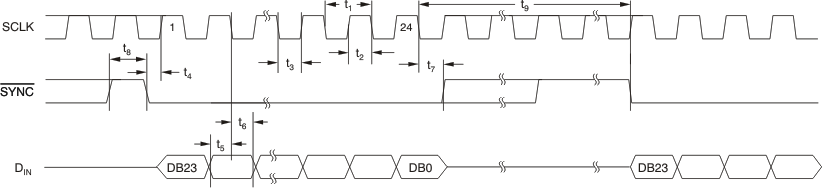Figure 1. Serial Write Operation

### 6.7 Typical Characteristics

#### 6.7.1 VDD = 5 V

at TA = 25°C, unless otherwise noted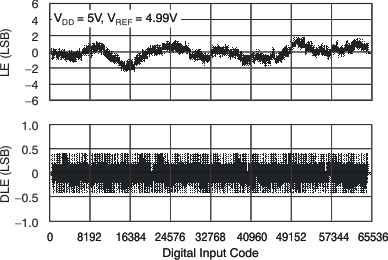Figure 2. Linearity Error and Differential Linearity Error
vs Digital Input Code (–40°C)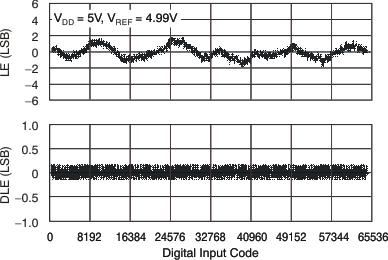Figure 4. Linearity Error and Differential Linearity Error
vs Digital Input Code (105°C)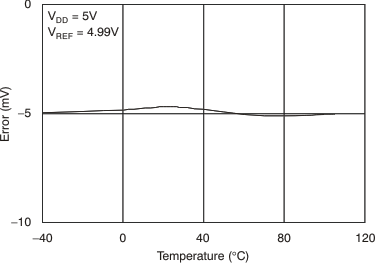Figure 6. Full-Scale Error vs Temperature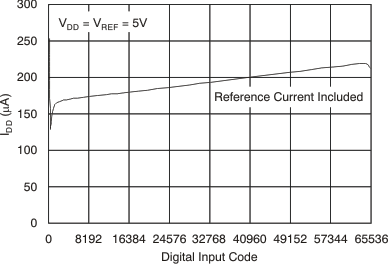Figure 8. Supply Current vs Digital Input Code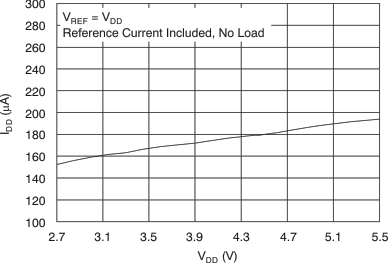Figure 10. Supply Current vs Supply VoltageFigure 12. Supply Current vs Logic Input Voltage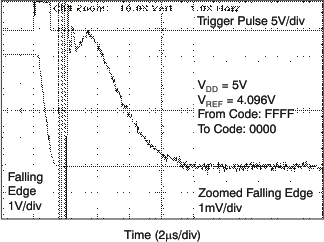5 V
Figure 14. Full-Scale Settling Time: Falling Edge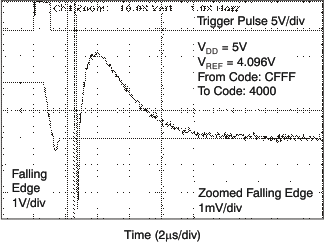5 V
Figure 16. Half-Scale Settling Time: Falling Edge5 V 1-LSB Step
Figure 18. Glitch Energy: Falling Edge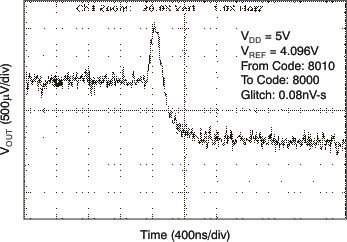5 V 16-LSB Step
Figure 20. Glitch Energy: Falling Edge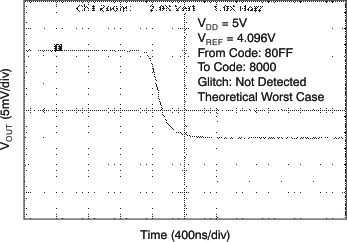5 V 256-LSB Step
Figure 22. Glitch Energy: Falling Edge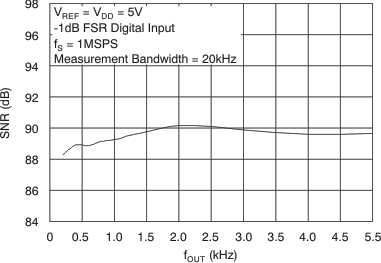Figure 24. Signal-to-Noise Ratio vs Output Frequency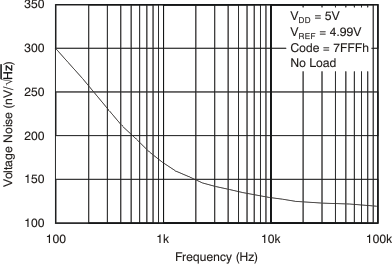Figure 26. Output Noise Density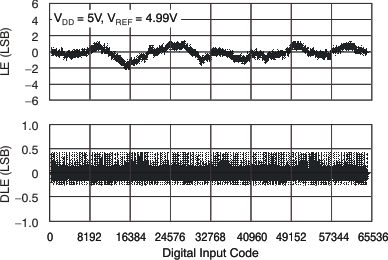Figure 3. Linearity Error and Differential Linearity Error
vs Digital Input Code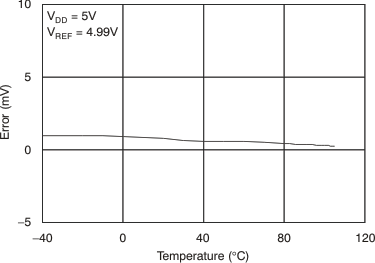Figure 5. Zero-Scale Error vs Temperature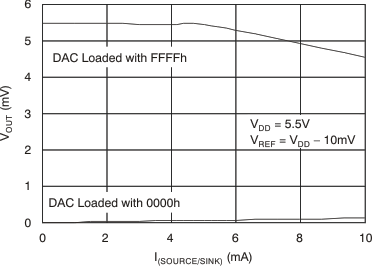Figure 7. Source and Sink Current Capability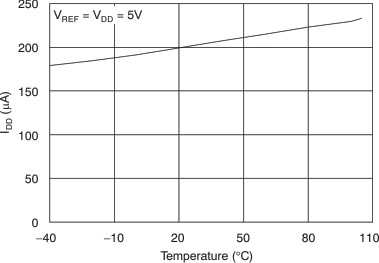Figure 9. Power-Supply Current vs Temperature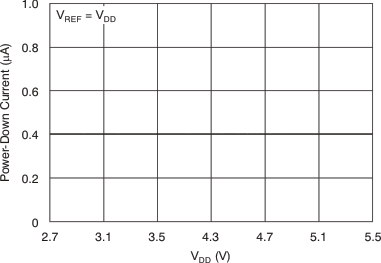Figure 11. Power-Down Current vs Supply Voltage5 V
Figure 13. Full-Scale Settling Time: Rising Edge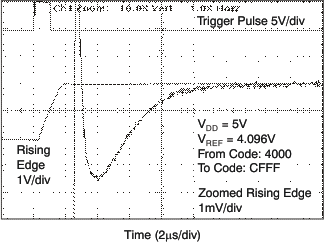5 V
Figure 15. Half-Scale Settling Time: Rising Edge5 V 1-LSB Step
Figure 17. Glitch Energy: Rising Edge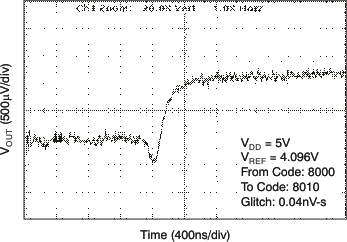5 V 16-LSB Step
Figure 19. Glitch Energy: Rising Edge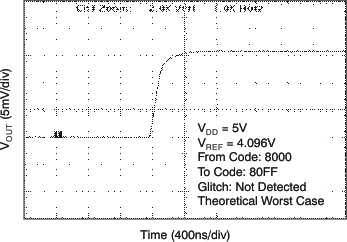5 V 256-LSB Step
Figure 21. Glitch Energy: Rising Edge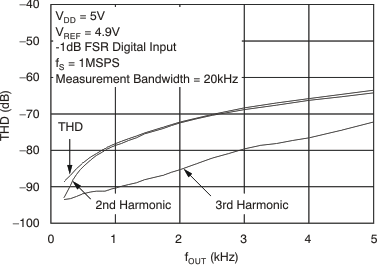Figure 23. Total Harmonic Distortion vs Output Frequency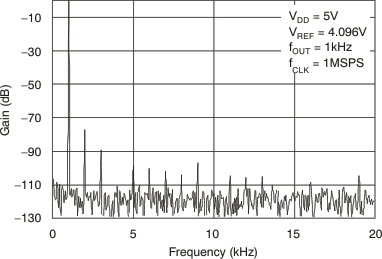Figure 25. Power Spectral Density

#### 6.7.2 VDD = 2.7 V

at TA = 25°C, unless otherwise noted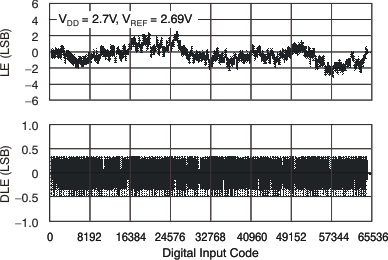Figure 27. Linearity Error and Differential Linearity Error
vs Digital Input Code (–40°C)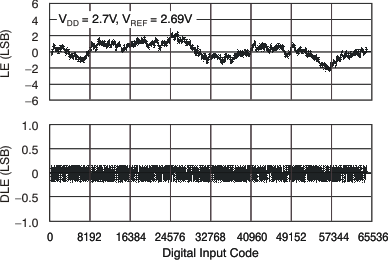Figure 29. Linearity Error and Differential Linearity Error
vs Digital Input Code (105°C)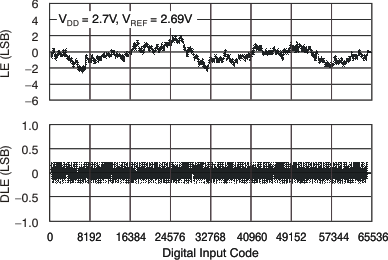Figure 28. Linearity Error and Differential Linearity Error
vs Digital Input Code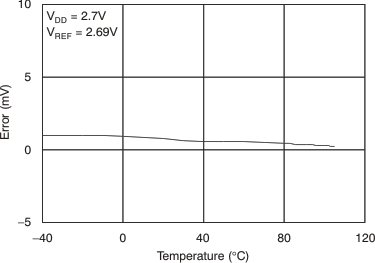Figure 30. Zero-Scale Error vs Temperature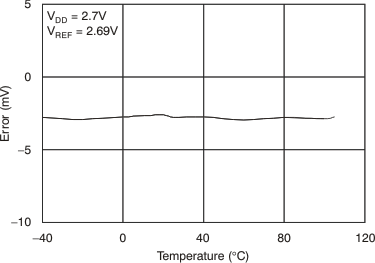Figure 31. Full-Scale Error vs Temperature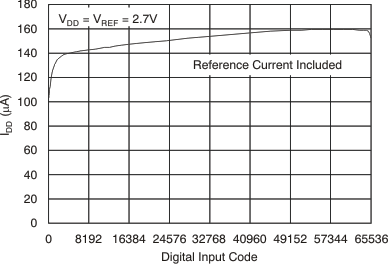Figure 33. Supply Current vs Digital Input CodeFigure 35. Supply Current vs Logic Input Voltage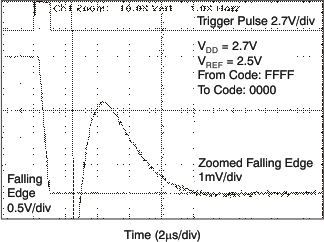2.7 V
Figure 37. Full-Scale Settling Time: Falling Edge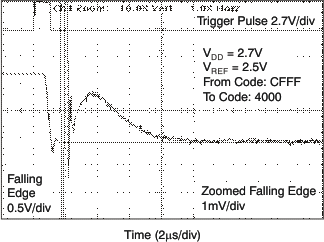2.7 V
Figure 39. Half-Scale Settling Time: Falling Edge2.7 V 1-LSB Step
Figure 41. Glitch Energy: Falling Edge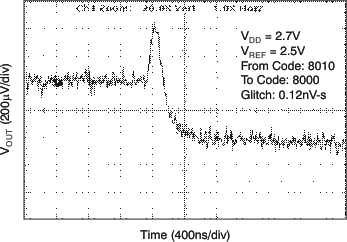2.7 V 16-LSB Step
Figure 43. Glitch Energy: Falling Edge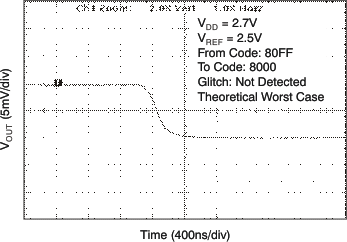2.7 V 256-LSB Step
Figure 45. Glitch Energy: Falling Edge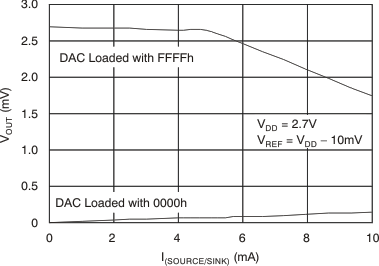Figure 32. Source and Sink Current Capability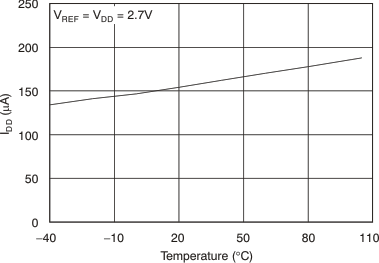Figure 34. Power-Supply Current vs Temperature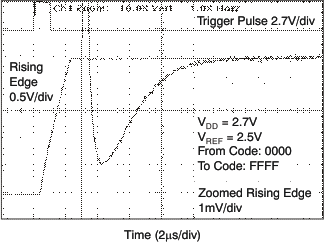2.7 V
Figure 36. Full-Scale Settling Time: Rising Edge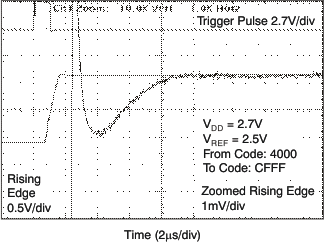2.7 V
Figure 38. Half-Scale Settling Time: Rising Edge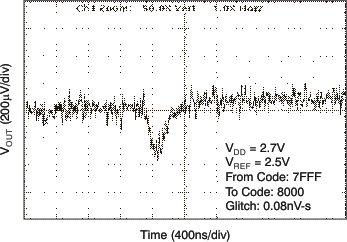2.7 V 1-LSB Step
Figure 40. Glitch Energy: Rising Edge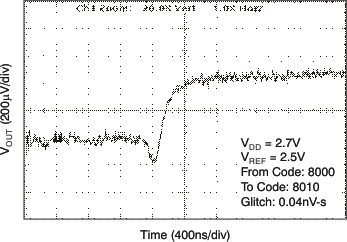2.7 V 16-LSB Step
Figure 42. Glitch Energy: Rising Edge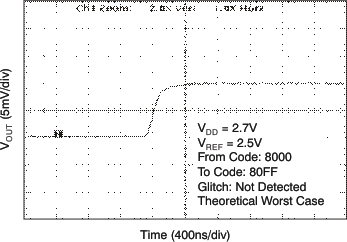2.7 V 256-LSB Step
Figure 44. Glitch Energy: Rising Edge